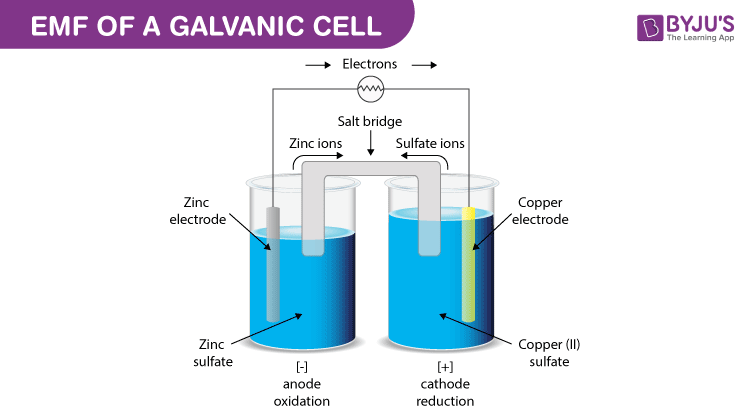# EMF of a cell & Cell notation

## What is EMF

EMF is abbreviated as the electromotive force which is defined as the maximum potential difference between the two electrodes of a voltaic or galvanic cell.

## EMF of a Galvanic cell## What is a galvanic cell

Galvanic cell is one of the most important electrochemical cells. A galvanic cell has a combination of two metal rods known as electrodes. Both the electrodes are immersed in a solution that contains the combination of ions and therefore form a half cell.

The half cells are connected by a salt bridge and the solution in which both the electrodes are dipped is known as the electrolyte. The chemical reaction happening in this cell is known as a redox reaction. Oxidation takes place in one of the electrodes that acts as an anode and reduction takes place on the other electrode that acts as a cathode.

## Cell notation

Some rules need to be taken care while representing an electrochemical cell. The cathode is on the right side and anode on the left side.

The cell is represented by the rule that metals are written first and then the metal ions that are present in the electrolyte. And these two need to be separated by a vertical line. For example Zn | Zn2+.

The molar concentration is written within the brackets as: Zn | Zn2+(1M)

If we take an example of Daniell cell, it can be represented as: Zn | Zn2+|| Cu2+ | Cu

Then cell notation is as follows:
Zn | Zn2+ (1M) || Cu2+ (1M)| Cu

## Cell potential or electromotive force (EMF)

It is the potential difference between electrodes in a galvanic cell and is measured in volts.

It can be represented as:
Emf of the cell= Half-cell potential at the cathode (right side) – Half-cell potential at the anode (left side).

## CellTo learn more about the determination of emf of a cell using Nernst Equation, Standard electrode potential , and more topics of chemistry, register with BYJU’S.

Test your knowledge on basic concepts of emf and cell notation!